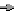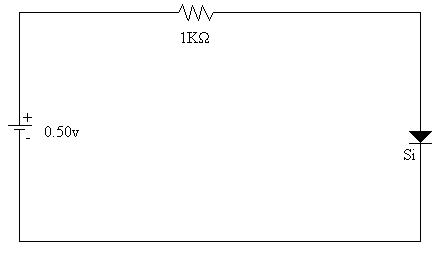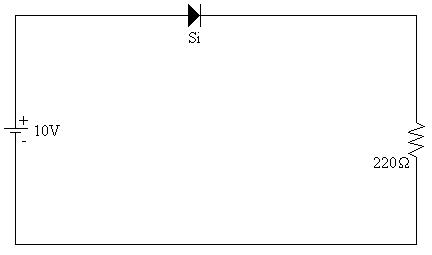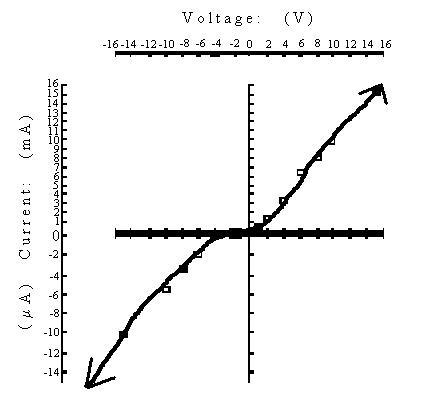A. Buettner

Page #6 of 6

EL - 200

Electronic Devices

Lab #1 The PN Diode

Andrew Buettner

March 6, 2003Objective

The objective of this lab is to study the voltage characteristics of a diode to draw it's diode curve.Equipment Used

1) ET Trainer

2) Multimeter

3) EL - 200 Lab kitProcedure

1) Measure the forward and reverse resistance of the diode

2) Assemble the following circuit:3) Measure the voltage across the resistor

4) Measure the current through the circuit

5) Reverse the diode, and change the resistor to 1MW and repeat steps 3 and 4

6) Repeat steps 3 through 5 changing the using the following voltages:

a) 1.0v

b) 2.0v

c) 4.0v

d) 6.0v

e) 8.0v

f) 10v

g) 15v

7) Assemble the following circuit8) Measure the voltage across the resistor

9) Measure the current through the circuit

10) Reverse the diode and repeat steps 8 and 9Data

A) Table 1: Forward and Reverse Biased Resistances:

Forward:

Reverse:

Resistance

18.5KW

'W

B) Table 2: Forward Biased Voltages and Currents:

Circuit voltage:

Diode:

.5v

1.0v

2.0v

4.0v

6.0v

8.0v

10v

15v

Voltage

.50v

.55v

.60v

.60v

.60v

.61v

.61v

.61v

Current

.065mA

.391mA

1.370mA

3.423mA

6.50mA

7.92mA

9.80mA

15.31mA

B) Table 2: Reversed Biased Voltages and Currents:

Circuit voltage:

Diode:

1.240v

2.0v

4.0v

6.0v

8.0v

10v

15v

Voltage

1.179v

1.814v

2.401v

2.606v

2.727v

2.819v

2.974v

Current

0.0000mA

0.0000mA

.0001mA

.0020mA

.0035mA

.0055mA

.0101mA

*Note: 1.240v in this circuit is the lowest attainable voltage*

C) Table 3: Forward vs. Reverse Bias for Schematic 2:

Forward Bias

Reverse Bias

Voltage:

9.31v

5.05v

Current:

44.7mA

24.24mAResults

A) Table 1: Forward Biased Results:

Circuit voltage:

Diode:

.5v

1.0v

2.0v

4.0v

6.0v

8.0v

10v

15v

Voltage

.50v

.55v

.60v

.60v

.60v

.61v

.61v

.61v

Current

.065mA

.391mA

1.370mA

3.423mA

6.50mA

7.92mA

9.80mA

15.31mA

Resistance

7.69KW

1.41KW

438W

175W

92W

77W

62W

40W

B) Table 2: Reversed Biased Results:

Circuit voltage:

Diode:

1.240v

2.0v

4.0v

6.0v

8.0v

10v

15v

Voltage

1.179v

1.814v

2.401v

2.606v

2.727v

2.819v

2.974v

Current

0.0000mA

0.0000mA

.0001mA

.0020mA

.0035mA

.0055mA

.0101mA

Resistance

'W

'W

24.01MW

1.303MW

779.1KW

510.9KW

294.5KW

*Note: 1.240v in this circuit is the lowest attainable voltage*

C) Diagram 1: Schematic 1 Enlarged view:D) Diagram 2: Schematic 2 Enlarged View:E) Diagram 3: Graph of Diode Curve:Answers To Lab Questions

1) Q: Plot the Voltage vs. Current for the diode:

A:2) Q: Calculate the bulk resistance for the diode:

A: The bulk resistance is:18.5KW.

3) Q: Why do the values of forward resistance and reverse resistance change as the diode's applied voltage change?

A: During forward biased, the diode has a constant voltage drop so it appears to be a variable resistance. During reversed bias, the diode has enough resistance as to let a nearly constant voltage through until it hits breakdown voltage.

4) Q: What approximations were used in this experiment and why?

A: The primary approximation made was that the resistor values were exact. Also, that the multimeters were accurate and did not influence the circuit's operation.Conclusions

From the data collected, ample information is given to draw a diode curve. The objective was therefore accomplished.Attachments

A) Original Handout

B) Original Lab Data

C) Calculations